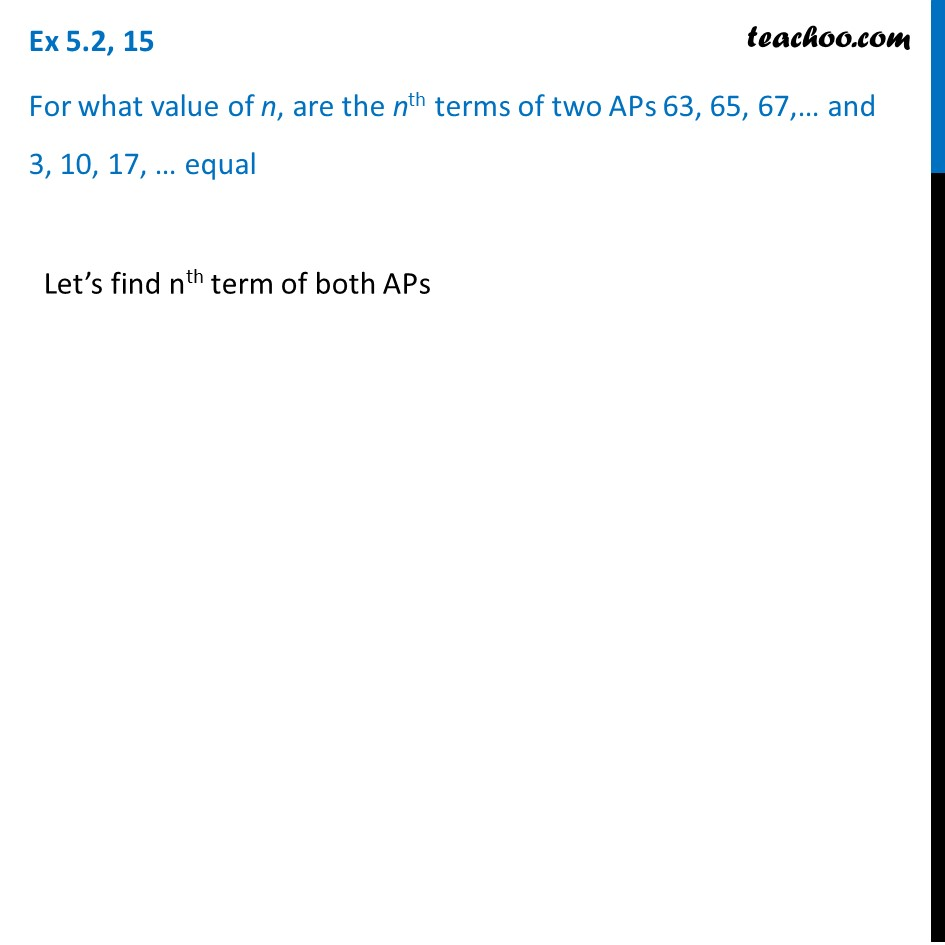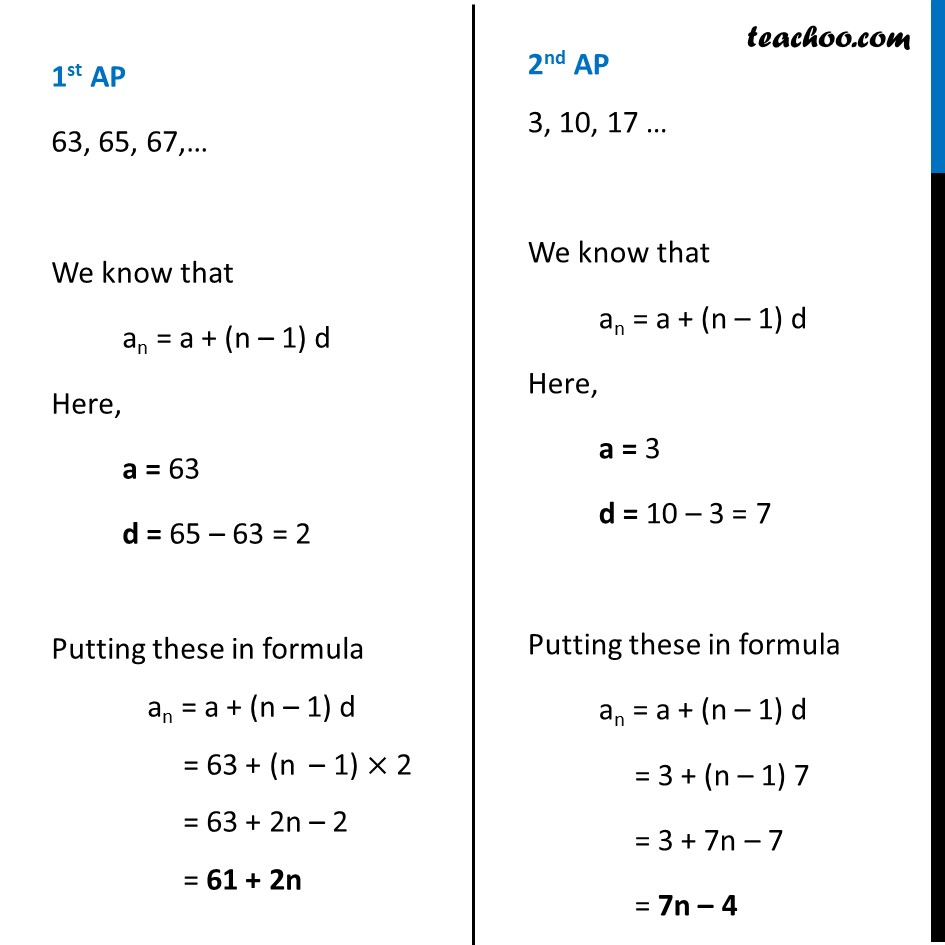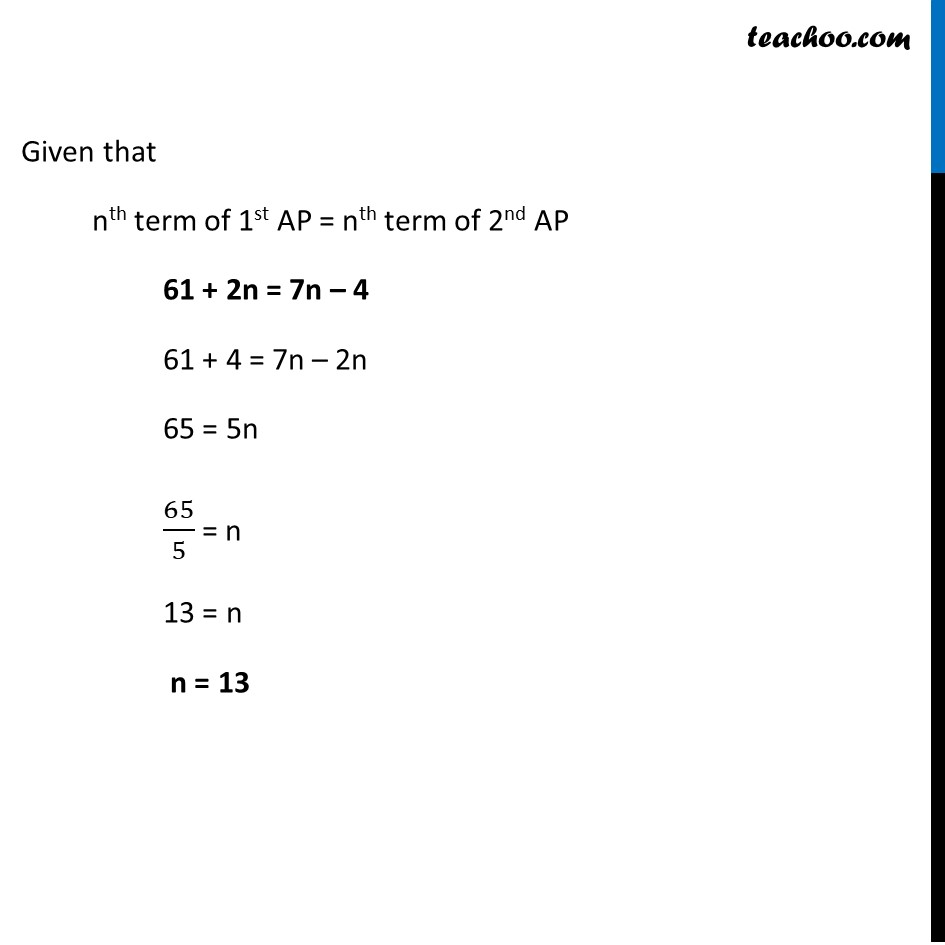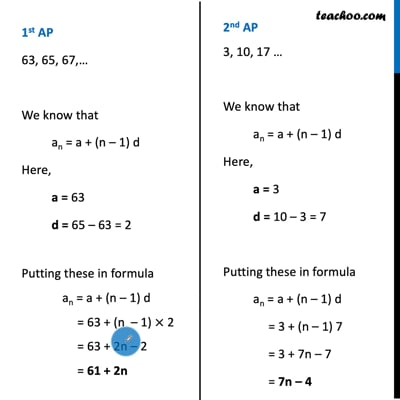Finding n

Chapter 5 Class 10 Arithmetic Progressions (Term 2)
Concept wiseThis video is only available for Teachoo black users

### Transcript

Ex 5.2, 15 For what value of n, are the nth terms of two APs 63, 65, 67,… and 3, 10, 17, … equal Let’s find nth term of both APs 1st AP 63, 65, 67,… We know that an = a + (n – 1) d Here, a = 63 d = 65 – 63 = 2 Putting these in formula an = a + (n – 1) d = 63 + (n – 1) × 2 = 63 + 2n – 2 = 61 + 2n 2nd AP 3, 10, 17 … We know that an = a + (n – 1) d Here, a = 3 d = 10 – 3 = 7 Putting these in formula an = a + (n – 1) d = 3 + (n – 1) 7 = 3 + 7n – 7 = 7n – 4 Given that nth term of 1st AP = nth term of 2nd AP 61 + 2n = 7n – 4 61 + 4 = 7n – 2n 65 = 5n 65/5 = n 13 = n n = 13